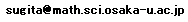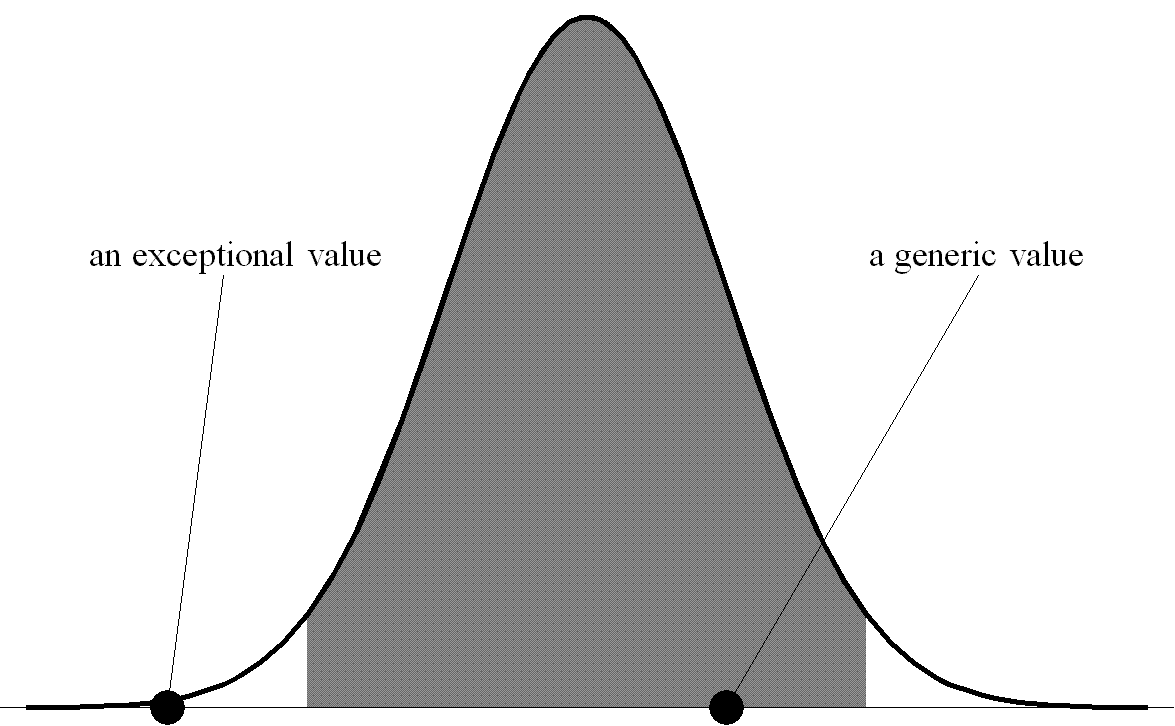Monte Carlo method, Random number, and Pseudorandom number

Hiroshi SUGITA Department of Mathematics, Graduate School of Science, Osaka UniversityWhat is the Monte Carlo method?
What is random number?
Why is random number necessary for the Monte Carlo method?
Can we really use pseudorandom number instead of random number? ...

Home

What is the Monte Carlo method?

The Monte Carlo method is a numerical method to solve mathematical problems by computer-aided sampling of random variables. For each individual problem, we set up a probability space --- for simplicity, we assume it to be a probability space of finite coin tosses

( {0,1}L, PL = the uniform probability measure), L N

--- and a random variable S defined on it, S:{0,1}LR. We evaluate S(ω) by a computer for some chosen ω{0,1}L, which procedure is called sampling.

The Monte Carlo method is a kind of gambling as its name indicates. The aim of the player, say Alice, is to get a generic value --- a typical value or not an exceptional value --- of S by sampling.Suppose that Alice has no idea which ω she should choose to get a generic value of S. She chooses an ω{0,1}L of her own will, realizing the risk of getting an exceptional value of S. Her risk is measured by the probability PL(A) of a set

A := { ω{0,1}L | S(ω) is an exceptional value of S }.

Since S seldom takes exceptional values, PL(A) is very small, and hence it seems easy for Alice to win this game.

What is random number?
Why is it necessary for the Monte Carlo method?

However, when L is very large, say L=100,000,000, it is not easy for her to win the game. To choose an ω{0,1}L, she cannot help using a computer because of its huge amount of information; ω is approximately a 12 MByte data. Obviously, a 12MByte data is too huge for anyone to input directly from a key board to a computer. So, she needs some device. But whatever device she may use, those ω's {0,1}L she can choose of her own will are very limited. Let us assume that Alice can input at most 1,000 bit data directly from the key board. Then the number of ω's {0,1}L she can choose is at most 21,001. (This is because the number of all the k bit data is 2k, and so the number of all data at most 1,000 bit is 21+22+...+21,000=21,001-2.) Since the number of all the elements of {0,1}100,000,000 is 2100,000,000, we see that how few those ω's {0,1}L she can choose are. By this reason, although PL(A) is very small, we cannot say that Alice is able to get a generic value of S almost certainly. Therefore, to let the risk evaluation PL(A) have a substantial meaning, it is natural to think that Alice needs an ω{0,1}L which she cannot choose of her own will --- Kolmogorov called such an ω a random number. This is the real reason why random number is needed for the Monte Carlo method.

Can we really use pseudorandom number instead of random number?

Is random number absolutely necessary for the Monte Carlo method? No, it is not. Pseudorandom number may suffice. A pseudorandom generator is a mapping which stretches short {0,1}-sequences into long {0,1}-sequences. For example, suppose that Alice uses a pseudorandom generator g:{0,1}n→{0,1}L, n< L, to sample S. First, she chooses a seed ω'{0,1}n of her own will. Here n should be so small that she may input ω' directly from a keyboard to a computer. Then the computer generates a pseudorandom number g(ω'){0,1}L from her seed ω', and she finally gets a sample S(g(ω')) of S. As a result, she is now betting on whether S(g(ω')) is a generic value of S or not. Her risk is now measured by the probability Pn(g(ω')A). This probability naturally depends on g, but if there exists a pseudorandom generator g such that Pn(g(ω')A) remains very small, Alice can actually get a generic value of S with high probability. Such a g is said to be secure against A. The problem of sampling in a Monte Carlo method is solved by finding a secure pseudorandom generator.

For a general random variable S, although there are many candidates, there is no pseudorandom generator which is proved to be secure against the set of those ω's{0,1}L that yield exceptional values of S. However, if we restrict the use of pseudorandom generator to the Monte Carlo integration, i.e., if S in question is a sample mean of i.i.d. random variables, there exists a pseudorandom generator which is secure against the set of those ω's{0,1}L that yield exceptional values of S. Such a pseudorandom generator has already been used in practice for not too large Monte Carlo integrations (Random Weyl sampling (RWS)).

H. SUGITA,
Probability and Random Number---A First Guide to Randomness,
World Scientific, pp.140.
http://www.worldscientific.com/worldscibooks/10.1142/10662

H. SUGITA,
Monte Carlo Method, Random Number, and Pseudorandom Number, MSJ Memoirs vol.25, (2011), pp.xiv+133.
Distribution: World Scientific